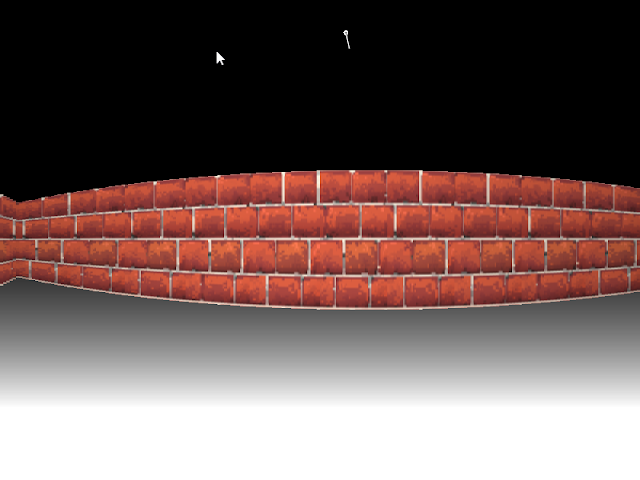### Project 4: Ray casting with a 2d graphics library.

Implementing this using one of my favorite frameworks (https://love2d.org/) in Lua was a lot of fun!

This is heavily inspired by Wolfenstein 3D.

It involved simple trigonometry, calculating ray/shape intersections, figuring out how to map textures and lighting effects.

A problem I encountered was a fisheye lens effect, because for each ray projected into the world, I would map the intersection distance directly to the height a wall (which is a group of line slices). However what I needed to do was calculate the perpendicular distance of the intersection using the projection plane as a reference.Fish-lens effect

Here's a great image which helped me understand the problem a lot, originally my raycasting method resembled the one of the far right.

Here are the resources I used:
• http://permadi.com/1996/05/ray-casting-tutorial-table-of-contents/ - Help with the projection plane/intersection distance calculation.
• https://en.wikipedia.org/wiki/Linear_interpolation - How I map the texture coordinates onto the side of a shape given a set of intersections with it.